Latest Banking jobs   »

# Quantitative Aptitude Quiz For Bank Foundation 2023 -19th April

Q1. If average of five consecutive odd numbers is 33, then the least odd number is:
(a) 27
(b) 31
(c) 29
(d) 23
(e) 33
Q2. The average weight of boys in a class of total strength 50 is 40 kg while average weight of girls is 35 kg. Find number of girls in class if average weight of class is 38 kg.
(a) 10
(b) 30
(c) 20
(d) 40
(e) 15

Q3. Three year ago, the average age of five employee of a company was 54 yrs. The present average age is 52 yr after the inclusion of a new employee entered. Find the age of the new employee?
(a) 18 yr
(b) 25 yr
(c) 20 yr
(d) 22 yr
(e) 27 yr

Q4. Average of four numbers is increased by 1 when a number is replaced by x and average of that four number is decreased by 1 when another number is replaced with x. What is the difference of the both numbers?
(a) 6
(b) 8
(c) 16
(d) 4
(e) Cannot be determined

Q5. 3 years ago, Average age of father and his son was 36 year. Present age of mother is 3 years less than twice of the age of son and ratio of mother’s age 3 years later to the father’s age 4 years ago is 24 : 25. Then, find the present average age of family ?
(a) 39 years
(b) 44 years
(c) 36 years
(d) 41 years
(e) 43 years

Q6. If 40% of (X + 2000) = 1300 and 60% of (X + Y) = 1830. Find X : Y .
(a) 5 : 8
(b) 20 : 27
(c) 8 : 13
(d) 26 : 35
(e) 25 : 36

Q7. If 40% of P is equal to 75% of Q then find 12P is what percent of 20Q?
(a) 120%
(b) 110%
(c) 107 ½%
(d) 100%
(e) 112 ½ %

Q8. The price of petrol is increased by 25% by what percent should a car owner reduce the consumption of petrol so that his expenditure on petrol remains constant?
(a) 18%
(b) 16%
(c) 15%
(d) 20%
(e) 14%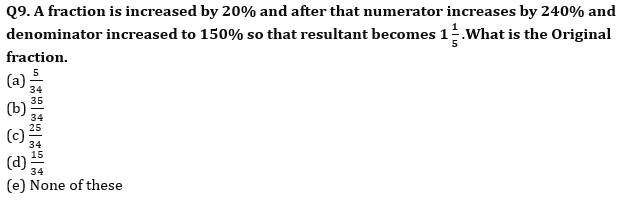Q10. 20% of (X + 2500) is equal to the 40% of (X + 900). Then find 50% of X ?
(a) 250
(b) 350
(c) None of these
(d) 450
(e) 150

Q11. The ratio of number of balls in bags x, y is 2 : 3. Five ball are taken from bag y and are dropped in bag x, number of balls are equal in each bag now. Number of balls in each bag now is
(a) 45
(b) 20
(c) 30
(d) 25
(e) 35

Q12. A and B earn in the ratio 2 : 1. They spend in the ratio 5 : 3 and save in the ratio 4 : 1. If the total monthly savings of both A and B are Rs. 5,000, the monthly income of B is
(a) Rs. 7,000
(b) Rs. 14,000
(c) Rs. 5,000
(d) Rs. 10,000
(e) Rs. 12,000

Q13. Ratio of two numbers is 5 : 7. If 30 is added in each number, the ratio becomes 3 : 4. Find the ratio of the number if 10 is subtracted from each number.
(a) 5 : 8
(b) 8 : 11
(c) 7 : 10
(d) 6 : 11
(e) 7 : 9

Q14. Two numbers A and B are in ratio 11 : 19, when 19 is added to A and 11 is added to B the ratio becomes 2 : 3, Find value of (20 + B)?
(a) 154
(b) 144
(c) 164
(d) 133
(e) 153

Q15. Ratio of income of A to that of B is 5:9. If expenditure of A is 3/8 th of his income and expenditure of B is 4/9 th of his income and sum of their saving is Rs 1950 then find the difference between their income?
(a) Rs 900
(b) Rs 1000
(c) Rs 880
(d) Rs 960
(e) Rs 920

Solutions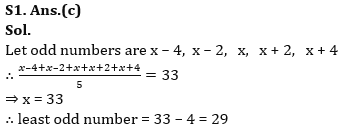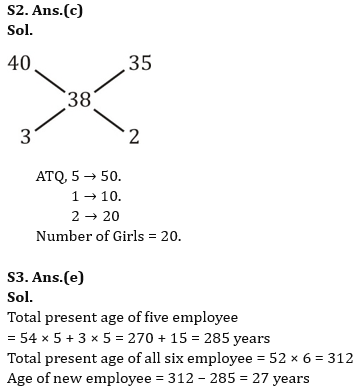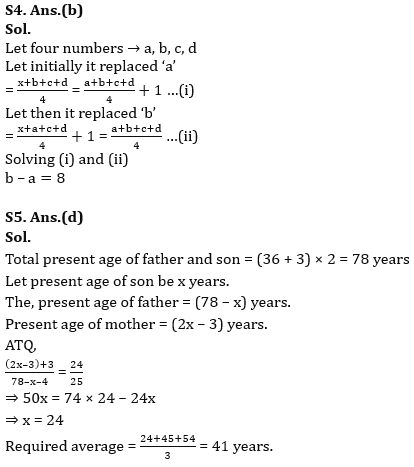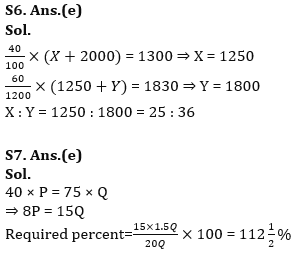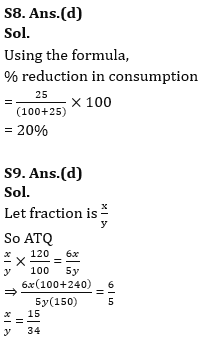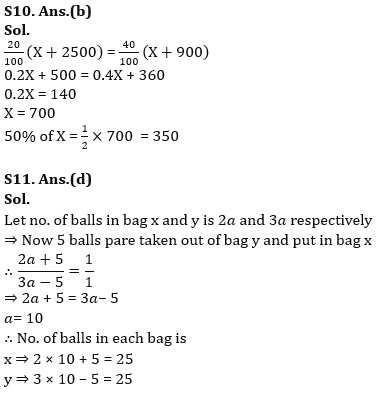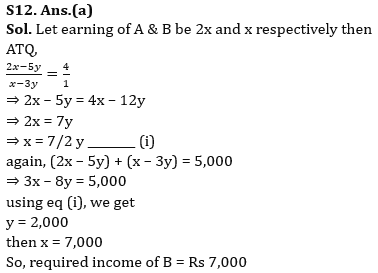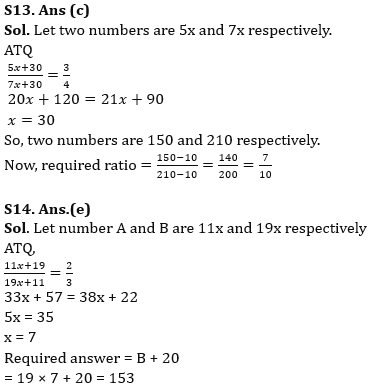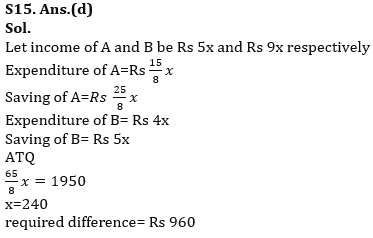## FAQs

### What is the selection process of the Bank Clerk?

The selection process of the Bank Clerk is Prelims & Mains.

#### Congratulations!Union Budget 2023-24: Free PDF